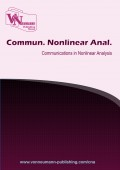# Solvability and asymptotic stability of a class of nonlinear functional-integral equation with feedback control

Volume 5, Issue 1, pp 19--27 Publication Date: 2018-08-13### Authors

Payam Nasertayoob - Department of Mathematics and Computer Science, Amirkabir University of Technology (Polytechnic), Hafez Ave., P. O. Box 15914, Tehran, Iran.

### Abstract

Using the technique of measure of noncompactness we prove the existence, asymptotic stability and global attractivity of a class of nonlinear functional-integral equation with feedback control. We will also include a class of examples in order to indicate the validity of the assumptions.

### Keywords

Functional integral equation, measure of noncompactness, asymptotic stability, feedback control, fixed point

### References

•  I. K. Aregon,, Quadratic equations and applications to Chandrasekhar’s and related equations,, Bull. Austral. Math. Soc., 32 (1985), 275–292. 1, 32 (1985), 275-292

•  J. Bana´s,, Measure of noncompactness in the space of continuous temperet functions, , Demonstratio Math., 14 (1981), 127–133

•  J. Bana´s, B. C. Dhage,, Global asymptotic stability of solutions of a functional integral equation,, Nonlinear Anal., 69 (2008), 1945–1952

•  J. Bana´s, K. Goebel,, Measure of noncompactness in the Banach space,, Dekker,, New York (1980),

•  J. Bana´s, B. Rzepka,, On existance and asymptotic stability of solutions of a nonlinear integral equation, J. Math. Anal. Appl., 284 (2003), 165–173

•  J. Bana´s, B. Rzepka, , An application of a measure of noncompactness in the study of asymptotic stability, App. Math. Lett., 16 (2003), 1-6

•  J. Bana´s, B. Rzepka,, On local attractivity and asymptotic stability of solutions of a quadatic Volterra integral equation,, Appl. Math. Comput., , 213 (2009), 102–111

•  J. Bana´s, D. O’Regan,, On existence and local attractivity of solutions of a quadratic Volterra integral equation of fractional order,, J. Math. Anal. Appl.,, 34 (2008), 573–582

•  F. Chen,, The permanence and global attractivity of Lotka-Volterra competition system with feedback control,, Nonlinear Anal., 7 (2006), 133–143, 7 (2006), 133-143

•  K. Deimling,, Nonlinear Functional analysis,, Springer-Verlag,, Berlin (1985),

•  Z. Liu, S. M. Kang, J. S. Ume,, Solvability and asymptotic stability of a nonlinear functional-integral equation, , App. Math. Lett., 24 (2011), 911–917, 24 (2011), 911–917